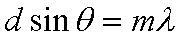## Diffraction Grating

Here is a model of a "force table spectroscope". Consider the top figure to the left - a top view of the spectroscope - the light comes in from the North, hits the diffraction grating, and then the light separates into the various colors. We are following 4 colors (approximately the spectrum of Helium) : Red = 677 nm, Yellow = 594 nm, Green = 500 nm, and Blue = 456 nm. You can see those beams radiate away from the diffraction grating.
But, we can also see that not all the colors of light show up (that is, the angle would be greater than 90 degrees) - so the bottom diagram shows the colors along an angle scale (0-90 degrees). We can now see something else, overlapping orders! To help us keep track of the orders, horizontal black lines are indicated between the 400-700 nm visible range.
Equation used is :where d = 1/spacing (in the correct units) ... thus distance between adjacent lines!
So, to put d into nanometers .... d=1000000/spacing_mm

Set the number of lines in the diffraction grating :
Spacing (lines/mm) =
(range limited to = 350-2250 lines/mm)
---------------------------------------------------
* If you manually change values ...

The grid below shows the angles for each of the colors - by order.

 Color\Order m=1 m=2 m=3 m=4 m=5 m=6 Red Yellow Green Blue

The top figure shows a top view of the "spectrometer" - the angles for which the light beams would exit the diffration grating (the black horizontal rectangle).

The figure on the bottom is an "spectrum plot" of the wavelengths vs the angles. Each order is "connected" with a line. Look for overlapping orders!